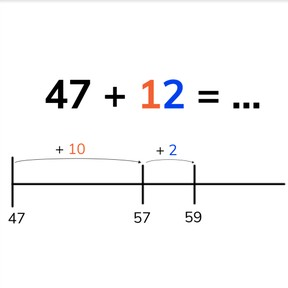Adding to 100 by tens and ones without regrouping

# Adding to 100 by tens and ones without regrouping

No account needed.8,000 schools use Gynzy92,000 teachers use Gynzy1,600,000 students use Gynzy

2.NBT.B.5
2.OA.A.1

## Relevance

Discuss with students that you can use adding by tens and ones when the numbers you are adding are too big to do at once in your head. An example would be if you take a train and there are 41 people on the train, and 26 more get on board.

## Introduction

Ask students to count by turns. Start using the given number and time how long it takes them to reach 100. Repeat this with a different starting number, and time them again. Ask students if they think they were faster the second time or the first. Next show students a number line with a few steps shown. Ask students what the total is.

## Development

Check that students understand adding to 100 by tens and ones by asking the following questions:
- What does it mean to add using tens and ones?
- What is the first step you take when you do this?
- How do you solve the problems 34+13 and 62+37?

## Guided practice

Students first practice adding with tens and ones with support of a number line. Then they calculate without the visual support.

## Closing

Discuss with students that you can use adding by tens and ones to make it easier to calculate larger addition problems. Ask students to solve a few exercises using the number line. They must write their own number lines and then compare them. By doing so, they can see that there are different ways to step to the answer. Finally students write the addition problems and solve the answers. Ask them to explain their thinking.

## Teaching tips

Students who have difficulty adding with tens and ones can make use of the number line. Have them practice splitting the second addend into tens and ones, and then making those steps on the number line. Emphasize that they first jump in tens, and then in ones on the number line.

### The online teaching platform for interactive whiteboards and displays in schools

• Save time building lessons

• Manage the classroom more efficiently

• Increase student engagement